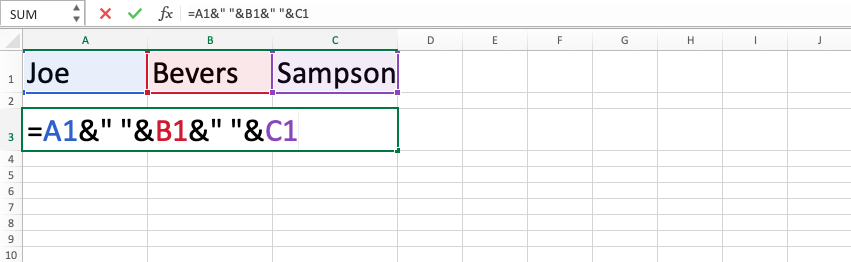CONCATENATE Function in Excel - Compute Expert

# CONCATENATE Function in Excel

Home >> Excel Tutorials from Compute Expert >> Excel Formulas List >> CONCATENATE Function in Excel

In this tutorial, how to use CONCATENATE in excel will be explained. CONCATENATE function in excel is useful to combine more than one data into one writing.

## Why do We Need to Learn About CONCATENATE in Excel?

In doing the processing of various kinds of data in a spreadsheet, sometimes we need to combine some of them to use the result as a cell content or for further analysis needs. If we manually do the combination, then it will be very difficult if there are much data to process. Besides, the chance for mistakes in the combining process will be higher.

The need for this combination can be found when we try to make a code for every data entry so it can be used as a marker or as an input for some formulas or perhaps, the simple example is when we need to combine front, middle, and last names to process name data in a spreadsheet. When the combination is done for those various kinds of needs but many data entries need this kind of process, then it will be impractical and take a lot of time if we do it manually.

Besides, if we do mistakes in the combination process because of carelessness when doing it manually, then it will not be good too for the thing that we want to show from the combination result or it can also cause an error if we input it in a formula that uses the combination result of more than one data as one of its input.

To help to solve problems like these, then you can use the CONCATENATE function in excel. CONCATENATE in excel is very useful when you need the combination process in a spreadsheet, especially when the process should be done many times.

## What is the CONCATENATE Function in Excel?

CONCATENATE function in excel is a function with the usage objective to get the combination result from more than one data. CONCATENATE in excel combines all of the inputs in sequence based on the input sequence in it and it will not give space between the inputs. Because of that, you need to add spaces on your own as its inputs if needed.

A bit explanation about the inputs in this formula is as follows:

=CONCATENATE(text1, text2, …)

Note:
text1, text2, … = all the data that you want to combine in one text

## How to Use CONCATENATE in Excel?

The next part will explain how to use the CONCATENATE function in excel. The formula of CONCATENATE in excel needs the input of all the things that you want to combine into one in the formula process.

## Using the CONCATENATE Function in Excel

1. Type equal sign ( = ) in the cell where you want to put the process result2. Type CONCATENATE (can be with large and small letters) and open bracket sign after =3. Type the data or cell coordinates where you put the things to be combined after open bracket sign with comma sign ( , ) between the data or cell coordinates4. Type close bracket sign after all inputs that you want to combine has been inputted5. Press Enter
6. The process is done!## Exercise

After you have learned CONCATENATE in excel completely, now is the time you practice your understanding through the exercise below!

### Questions

Use CONCATENATE function in excel to answer each question!
1. Create a code for each product by using the name, sales region, and priority!
2. Create a code for each product by using the name, group, and sales priority!
3. Create a code for each product by using the name, sales region, group, and sales priority! Use - as separation signs between each data in the combination result!

• If you input a data as one of the formula input, then it will use the serial number of the date in the combination result
• You can also use & sign between the things that you want to combine to produce the same combination result as CONCATENATE in excelGet updated excel info from Compute Expert by registering your email. It's free!